Angle + similarity of triangles - examples

1. RailwaysRailways climb 7.4 ‰. Calculate the height difference between two points on the railway distant 3539 meters.
2. SimilarityAre two right triangles similar to each other if the first one has a acute angle 70° and second one has acute angle 20°?
3. ClimbOn the road sign, which informs the climb is 8.7%. Car goes 5 km along this road. What is the height difference that car went?
4. ClimbRoad has climbing 1:27. How big is a angle corresponds to this climbing?
5. Boat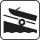A force of 300 kg (3000 N) is required to pull a boat up a ramp inclined at 14° with horizontal. How much does the boat weight?
6. Sun raysIf the sun's rays are at an angle 60° then famous Great Pyramid of Egypt (which is now high 137.3 meters) has 79.3 m long shadow. Calculate current height of neighboring chefren pyramid whose shadow is measured at the same time 78.8 m and the current hei
7. CosineCalculate the cosine of the smallest internal angle in a right-angled triangle with cathetus 3 and 8 and with the hypotenuse 8.544.Tree perpendicular to the horizontal surface has a shadow 8.32 meters long. At the same time meter rod perpendicular to the horizontal surface has shadow 64 cm long. How tall is tree?
9. Diagonal in rectangle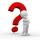In that rectangle ABCD is the center of BC point E and point F is center of CD. Prove that the lines AE and AF divide diagonal BD into three equal parts.
10. Angle in RTDetermine the size of the smallest internal angle of a right triangle whose sides constitutes sizes consecutive members of arithmetic progressions.
11. GarageThere are two laths in the garage opposite one another: one 2 meters long and the second 3 meters long. They fall against each other and stay against the opposite walls of the garage and both laths cross 70 cm above the garage floor. How wide is the garag
12. Area and two anglesCalculate the size of all sides and internal angles of a triangle ABC, if it is given by area S = 501.9; and two internal angles α = 15°28' and β = 45°.
13. RhombusABCD is a rhombus, ABD is an equilateral triangle and AC is equal to 4. Find the area of the rhombus.
14. Traffic laws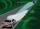Under traffic regulations, car lights can illuminate the road up to a maximum of 30 m. To check the reach of the dipped-beam lights of their car, Peter stopped car at 1.5 m from the wall. The dipped-beam headlights are 60 cm high. At what height on the wa
15. V-belt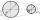Calculate a length of the V-belt when the diameter of the pulleys is: D1 = 600 mm D2 = 120 mm d = 480 mm
16. Mirror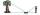How far must Paul place a mirror to see the top of the tower 12 m high? The height of Paul's eyes above the horizontal plane is 160 cm and Paul is from the tower distant 20 m.
17. Ruler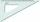How far from Peter stands 2m hight John? Petr is looking to John over ruler that keeps at arm's distant 60 cm from the eye and on the ruler John measured the height of 15 mm.
18. Hexagon cut pyramidCalculate the volume of a regular 6-sided cut pyramid if the bottom edge is 30 cm, the top edge us 12 cm, and the side edge length is 41 cm.
19. Area of iso-trapFind the area of an isosceles trapezoid, if the lengths of its bases are 16 cm, and 30 cm, and the diagonals are perpendicular to each other.Miro stands under a tree and watching its shadow and shadow of the tree. Miro is 180 cm tall and its shade is 1.5 m long. The shadow of the tree is three times as long as Miro's shadow. How tall is the tree in meters?